## research papersJOURNAL OFSYNCHROTRONRADIATION
ISSN: 1600-5775
Volume 26| Part 4| July 2019| Pages 1069-1072

## Diffraction properties of a strongly bent diamond crystal used as a dispersive spectrometer for XFEL pulses1

aEuropean X-ray Free-Electron Laser Facility, Holzkoppel 4, D-22869 Schenefeld, Germany, bESRF – The European Synchrotron, CS40220, 38043 Grenoble Cedex 9, France, cPaul-Drude-Institut für Festkörperelektronik, Hausvogteiplatz 5–7, 10117 Berlin, Germany, and dTISNCM - Technological Institute for Superhard and Novel Carbon Materials, 142190 Moscow, Russia
*Correspondence e-mail: liubov.samoylova@xfel.eu

(Received 7 January 2019; accepted 9 April 2019; online 6 June 2019)

Self-amplified spontaneous emission (SASE) enables X-ray free-electron lasers (XFELs) to generate hard X-ray pulses of sub-100 fs duration. However, due to the stochastic nature of SASE, the energy spectrum fluctuates from pulse to pulse. Many experiments that employ XFEL radiation require the resolution of the spectrum of each pulse. The work presented here investigates the capacity of a thin strongly bent diamond crystal to resolve the energy spectra of hard X-ray SASE pulses by studying its diffraction properties. Rocking curves of the symmetric C*(440) reflection have been measured for different bending radii. The experimental data match the theoretical modelling based on the Takagi–Taupin equations of dynamical diffraction. A uniform strain gradient has proven to be a valid model of strain deformations in the crystal.

### 1. Introduction

The stochastic nature of self-amplified spontaneous emission (SASE) radiation (Saldin et al., 1998) requires to effectively resolve the spectral structure of each pulse for a number of experimental techniques at X-ray free-electron lasers (XFELs), including imaging and spectrometry. Previously, a spectrometer based on an elliptical mirror and a flat crystal in Bragg reflecting geometry has been successfully used to resolve SASE spectra at the SPring-8 Å Compact Free-Electron Laser (SACLA) in Japan (Inubushi et al., 2012). However, since the whole pulse is reflected by the mirror, this device cannot be used for experiments where in-line diagnostics is required. One option for in-line spectroscopy of SASE pulses is to extract a small fraction of the beam by a focusing diamond grating and use it for spectral analysis, while passing the zeroth order to the experiment (Karvinen et al., 2012).

Strongly bent crystals inserted in the direct beam can be used as in-line spectrometers for SASE radiation. In the case of the finite spectral bandwidth of an incident pulse, Bragg's condition 2d sin θ = λ is satisfied for a range of wavelengths at different points on the bent crystal surface, because within the beam the X-rays impinge at different angles. Previously, strongly bent thin silicon crystals have been successfully used as spectrometer devices for hard X-ray SASE pulses at the Linac Coherent Light Source (LCLS) in the USA (Zhu et al., 2012; Rich et al., 2016) and for soft X-ray pulses at the SwissFEL (Rehanek et al., 2018). Also, a bent crystal spectrometer can be placed in a diffraction order of a transmission grating, which works as a splitter. This technique has been demonstrated at the LCLS, using a diamond grating with a 0.2 µm pitch (Makita et al., 2015), and at SACLA, using a silicon grating with a 0.5 µm pitch (Katayama et al., 2016). Compared with a bent silicon crystal inserted directly in the XFEL beam, this technique allows for higher X-ray transmission. However, to use the grating's first diffraction order for spectral analysis and provide a sufficient offset from the direct beam, the bent silicon crystal has to be placed at a distance of several metres after the grating, which is not always possible.

The European XFEL generates sub-100 fs long pulses at an extremely high repetition rate of up to 4.5 MHz, with an estimated peak power of 20 GW (Altarelli et al., 2007). According to simulations (Saldin et al., 1999), the pulse-averaged radiation fluence can reach values of ∼0.4 J mm−2 in the hard X-ray regime. Under such intense radiation, the diffraction properties of silicon can change significantly due to lattice deformations caused by heating and the crystal can be damaged in cases of continuous exposure. For this reason, diamond is the preferred material for an in-line non-destructive spectrometer. Compared with silicon, the heat conductivity of diamond is more than ten times higher (Ho et al., 1972), and carbon absorption at hard X-ray wavelengths is an order of magnitude lower (Henke et al., 1993). Thus, diamond can be used as a compact in-line spectrometer for high-intensity hard X-ray SASE pulses.

The spectrometer is based on a 20 µm-thick diamond crystal and is dedicated to high repetition rate XFELs. In a previous experiment at the LCLS with a bent diamond and a bent silicon spectrometer mounted in tandem, the diamond spectrometer showed a similar if not superior performance (Boesenberg et al., 2017).

Prior to the future application of our device to SASE pulses at the European XFEL, in this work we study the diffraction properties of strongly bent ultra-thin crystals. We calculate the dynamical diffraction of a narrow beam incident on a thin crystal with a constant strain gradient. Rocking curves of a bent diamond crystal acquired experimentally for various bending radii are compared with the calculated curves.

### 2. Experimental

The 20 µm thin diamond crystal and the slider are fixed on a base plate of chemical vapour deposition (CVD) diamond. The thin crystal plate was cut from a defect-free high-pressure high-temperature (HPHT) type IIa synthetic diamond crystal. The crystal was additionally mechanically polished to a thickness of 20 µm. A tip that is fixed on the slider presses on the crystal. A linear translation of the slider enables the bending radius of the crystal to be changed (for details see Terentyev et al., 2016). The translation range was designed to allow for bending radii between 9.5 and 6.5 cm.

Rocking curve experiments were performed on the Nuclear Resonance Beamline ID18 (Rüffer & Chumakov, 1996) at the ESRF. A high-heat-load cryogenically cooled Si(111) monochromator reduced the energy bandwidth down to 2.1 eV, with subsequent monochromatization down to 0.5 meV bandwidth using a high-resolution four-bounce silicon monochromator. Such precise tuning allows for small steps during energy scans of the crystal diffraction. Slits were used to reduce the beam size on the sample to about 10 × 50 µm (vertical × horizontal). For the used photon energy of 14.4 keV, a 10 µm2 slit causes an additional angular divergence of about 10 µrad. The sample was mounted on a high-precision six-circle diffractometer (Huber) with additional horizontal and vertical translations for precise alignment to the centre of rotation. The diffracted signal was detected using an avalanche photo diode (APD) (Baron, 2000). Rocking curves were obtained by scanning the incident energy using the high-resolution monochromator. Due to the narrow bandwidth of the combination of both monochromators, the central energy had to be stepwise re-adjusted to cover the full width of the rocking curve of the spectrometer. Additionally, rocking curves of the bent crystal were measured at different bending radii.

### 3. Calculation of the diffraction curves

X-ray diffraction from elastically bent crystals has been the subject of detailed theoretical studies. Analytical solutions were obtained by Chukhovskii & Petrashen' (1977), Chukhovskii et al. (1978) and Chukhovskii & Malgrange (1989), and supplemented with numerical simulations by Gronkowski & Malgrange (1984) and Gronkowski (1991). These studies considered diffraction of an incident plane wave in a crystal with a thickness much larger than the extinction length, so that bending radii as large as hundreds of metres already resulted in a notable broadening of the Darwin diffraction curve. The results of such studies are not directly applicable to our case of thin crystals. However, the diffracted intensity can be calculated by the same approach as used by Gronkowski & Malgrange (1984) and Gronkowski (1991), namely, by numerical solution of the Takagi–Taupin equations of dynamical diffraction in a crystal with a strain gradient.

Solution of the diffraction problem requires knowledge of the displacement field in a bent crystal. Since our study is restricted to the symmetrical Bragg case, the displacement component uz(x, z), where the x axis is along the surface in the diffraction plane and the z axis is along the surface normal, needs to be determined. The bending axis is the y axis normal to the scattering plane. An accurate calculation of the displacement requires accounting for the elastic anisotropy of the crystal (Chukhovskii et al., 1994, 1996). For a cubic crystal bent by moment about the y axis, the displacement field in the plane y = 0 is [Lekhnitskii (1981), equation (16.3)],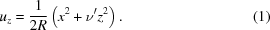Here, R is the bending radius defined by the applied moment,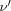=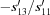is the Poisson ratio describing the strain in the z direction arising as a response to the strain applied in the x direction (Wortman & Evans, 1965) and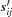are the components of the elastic compliances tensor. The prime denotes the tensor components referring to the actual crystal orientation. They can be calculated by rotating the compliances tensor from the standard 〈100〉 orientation to the one under consideration (Wortman & Evans, 1965). For the elastic constants of diamond (McSkimin & Andreatch, 1972), with the bending axis (y axis)  and surface orientation (z axis) , we find= 0.008, i.e. the Poisson effect is extremely small. For comparison, the elastic constants of silicon for the same directions give rise to= 0.06.

We calculate the dynamical diffraction from a bent crystal with the displacement field of equation (1) by a numerical solution of the Takagi–Taupin equations based on the algorithm proposed by Authier et al. (1968). The incident wave front is restricted to a width of 10 µm by the entrance slits. The symmetric Bragg reflection 440 of monochromatic 14.4 keV X-rays on a 20 µm thick diamond crystal is considered. Fig. 1shows the calculated spatial distribution of diffracted intensity at the surface of the crystal bent to a radius of 6.5 cm for different orientations of the incident beam, at the centre of the diffraction curve (black line, δθ = 0) and for angular deviations δθ = ±0.15 mrad from it (red and blue lines).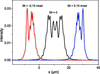Figure 1 Dynamical diffraction simulation of the spatial distribution of diffracted intensity at the surface of the crystal. The curves are calculated by numerical solution of the Takagi–Taupin equations for a radius of curvature of 6.5 cm, with the x axis along the surface of the bent crystal. The incident beam direction is taken at the maximum of the rocking curve (δθ = 0, black curve) and for angular deviations δθ = ±0.15 mrad from it (red and blue curves). The entrance slit is 10 µm wide and the crystal is 20 µm thick.

The diffracted wave evolves during further propagation in vacuum from the crystal to the detector. In the far field, it becomes a Fourier transformation of the spatial distribution shown in Fig. 1. In our experiment, the integrated intensity of this wave is collected by a detector. The angular integration at the detector gives, by Parseval's theorem, the same integrated intensity as the spatial integration at the crystal surface. This integrated intensity I(δθ), as a function of the angular deviation of the incident beam δθ, is the rocking curve of a bent crystal. The rocking curves calculated for different curvature radii are presented in Figs. 2 and 3 (solid lines). As expected, the rocking curve broadens with decreasing bending radius.

### 4. Results and discussion

Rocking curves acquired with energy scans are shown in Fig. 2for two selected bending radii. The experimental data match the modelled curves very well, indicating that the dynamical diffraction calculations for a model crystal with a uniform strain gradient are valid.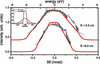Figure 2 Experimentally measured (grey) and theoretical (red) rocking curves for bending radii of 6.5 and 9.5 cm. For comparison, in the inset the calculated rocking curves for a 20 µm-thick flat diamond crystal are shown; the solid red line corresponds to the intrinsic rocking curve and the dotted line corresponds to the rocking curve taking into account the experimental angular divergence. For the energy-scan data, the angular deviation is calculated by the differential Bragg equation δθ = tan θ (ΔE/E). The FWHMs of the rocking curves are 0.326 mrad (5.02 eV) and 0.224 mrad (3.46 eV) for bending radii of 6.5 and 9.5 cm, respectively. In the theoretical rocking curves, the corresponding absolute values of peak intensity are 0.004 and 0.006 for a plane incident wave limited by a slit 10 µm wide.

The noise in the experimental data is due to the narrow bandwidth of the combined monochromators, requiring stepwise adaptation of the central energy. Moreover, the tails of the experimental curves demonstrate an asymmetry, which may be due to inhomogeneity of the applied strain. Measurements over a wider energy range will give more detailed information about the strain gradient.

It has been shown that diamond can withstand hard X-ray SASE radiation without significant damage for fluences up to ∼0.59 J mm−2 at 8.2 keV photon energy (Uhlén et al., 2013). In the case of intense pulses with high repetition rates, one should also consider the emerging transient thermal strain waves. It has been shown that the impact of these waves can become critical for ultra-thin diamond crystals with thickness of ∼10 µm and repetition rates of 100 kHz and above (Yang et al., 2018).

Rocking curves measured with θ scans are shown in Fig. 3. The curves are slightly wider than modelled, which is presumably due to imperfect alignment of the spectrometer to the beam spot. If the centre of rotation does not coincide exactly with the intersection of the beam and crystal surface, then during rotation of a bent crystal the beam illuminates different points of the surface. In this case, compared with rotation around a fixed point of the crystal, the same rotation angle results in a smaller change in the incidence angle, which leads to rocking curve broadening. For larger bending radii, this effect is small, and the widths of the measured rocking curves are closer to the theoretical ones.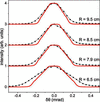Figure 3 Rocking curves measured with θ scans (black lines with dots) and theoretical rocking curves (solid lines) for various bending radii (indicated for each curve). The FWHMs of the rocking curves are 0.27 and 0.25 mrad for bending radii of 7.9 and 8.5 cm, respectively. For weaker bending (larger bending radii), the experimental rocking curves match the theory better.

### 5. Conclusion and outlook

We have studied the diffraction properties of a strongly bent diamond crystal spectrometer designed for hard X-ray SASE pulses at the European XFEL. The small radius of curvature of the crystal allows for a wide range of incidence angles to be reflected within a given beam size, i.e. the rocking curve of a bent crystal is much wider than that of a flat crystal. A numerical solution of the Takagi–Taupin equations for a crystal with a uniform strain gradient has been used to calculate the X-ray diffraction in the bent crystals.

The measured rocking curves match the calculated curves, which indicates that the uniform strain-gradient model is valid for the distortions in a thin strongly bent crystal. This model of crystal lattice deformation will be used elsewhere for a full-scale theoretical analysis of the angular resolution of such spectrometers and for the modelling of SASE pulse diffraction in strongly bent crystals.

### Footnotes

1This article will form part of a virtual special issue containing papers presented at the PhotonDiag2018 workshop.

### AcknowledgementsJOURNAL OFSYNCHROTRONRADIATION